Want to share your content on R-bloggers? click here if you have a blog, or here if you don't.

10.09.2015

Here I present a summary of a small spatstat workshop I created. I also explain in several videos how to transfer and convert 2d and 3D ImageJ measurements to a spatial point pattern with Bio7. The example datasets and code samples used here were taken from the spatstat help and from spatstat scripts cited at the end of the summary. The github repository with spatstat scripts, image scripts, documentation and more can be found here. I hope that this material is useful for others and helps in the creation of a spatial point pattern analysis with the fantastic spatstat package.

## Spatstat

From the spatstat website:

‘spatstat is a package for analyzing spatial point pattern data. Its functionality includes exploratory data analysis, model-fitting, and simulation.’ (1)

The package supports:

• creation, manipulation and plotting of point patterns
• exploratory data analysis
• simulation of point process models
• parametric model-fitting
• hypothesis tests and model diagnostic

## Classes in spatstat

To handle point pattern datasets and related data, the spatstat package supports the following classes of objects:

• ppp: planar point pattern
• owin: spatial region (‘observation window’)
• im: pixel image
• psp: pattern of line segments
• tess: tessellation
• pp3: three-dimensional point pattern
• ppx: point pattern in any number of dimensions
• lpp: point pattern on a linear network

## Creation of a point pattern object

A point pattern object can be created easily from x and y coordinates.

``````library (spatstat)
# some arbitrary coordinates in [0,1]
x <- runif(20)
y <- runif(20)
# the following are equivalent
X <- ppp(x, y, c(0,1), c(0,1))
X <- ppp(x, y)
X <- ppp(x, y, window=owin(c(0,1),c(0,1)))
# specify that the coordinates are given in metres
X <- ppp(x, y, c(0,1), c(0,1), unitname=c("metre","metres"))
``````## Study region (window)

Many commands in spatstat require us to specify a window, study region or domain!

An object of class “owin” (‘observation window’) represents a region or window (rectangular, polygonal with holes, irregular) in two dimensional space.

Example:

``````w <- owin(c(0,1), c(0,1))
# the unit square
w <- owin(c(10,20), c(10,30), unitname=c("foot","feet"))
# a rectangle of dimensions 10 x 20 feet
# with lower left corner at (10,10)
``````

``````# polygon (diamond shape)
w <- owin(poly=list(x=c(0.5,1,0.5,0),y=c(0,1,2,1)))
w <- owin(c(0,1), c(0,2), poly=list(x=c(0.5,1,0.5,0),y=c(0,1,2,1)))
# polygon with hole
ho <- owin(poly=list(list(x=c(0,1,1,0), y=c(0,0,1,1)),
list(x=c(0.6,0.4,0.4,0.6), y=c(0.2,0.2,0.4,0.4))))
``````

``````plot(w)
``````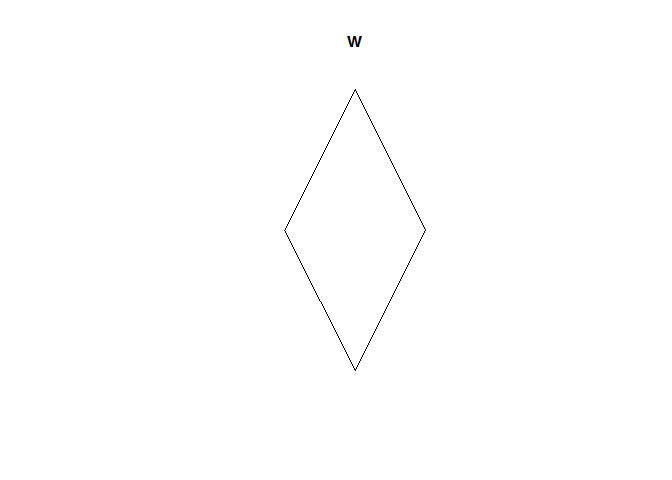``````plot(ho)
``````# Example dataset

``````data(swedishpines)
X<-swedishpines
plot(X)
``````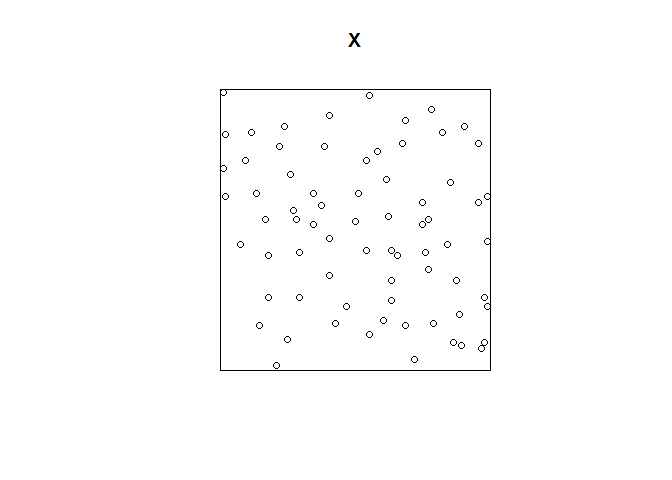Summary statistics of the dataset

``````summary(X)

## Planar point pattern:  71 points
## Average intensity 0.007395833 points per square unit (one unit = 0.1
## metres)
##
## Coordinates are integers
## i.e. rounded to the nearest unit (one unit = 0.1 metres)
##
## Window: rectangle = [0, 96] x [0, 100] units
## Window area = 9600 square units
## Unit of length: 0.1 metres
``````

Computes the distance from each point to its nearest neighbour in a point pattern.

``````nearest<-nndist(X)
summary(nearest)

##    Min. 1st Qu.  Median    Mean 3rd Qu.    Max.
##   2.236   5.541   8.246   7.908  10.050  15.650
``````

Finds the nearest neighbour of each point in a point pattern.

``````data(cells)
m <- nnwhich(cells)
m2 <- nnwhich(cells, k=2)
b <- cells[m]
plot(cells)
arrows(cells\$x, cells\$y, b\$x, b\$y, angle=15, length=0.15, col="red")
``````Density plot:

``````plot(density(swedishpines, 10))
``````Contour plot of the dataset

``````contour(density(X,10), axes=FALSE)
``````## How to create point data from an ImageJ particle analysis and Bio7

1. Open an image dataset (we open the blobs.gif example image from the internet)
2. Threshold the image
3. Make a Particle Analysis (Analyze->Analyze Particles…). Select the option ‘Display results’
4. Transfer the Results table data with the Image-Methods view action ‘Particles’ (ImageJ-Canvas menu: Window->Bio7-Toolbar – Action: Particles)
5. Execute the R script below

``````library(spatstat)
imageSizeX<-256
imageSizeY<-254
#Plot is visualized in ImageJ (Java) coordinates!
X<- ppp(Particles\$X, Particles\$Y, c(0,imageSizeX), c(0,imageSizeY))
plot(x = 1, y = 1,xlim=c(0,imageSizeX),ylim=c(imageSizeY,0), type = "n", main = "blobs.gif", asp = 1, axes = F, xlab = "x", ylab = "y")
axis(1)
axis(2, las = 2)
``````We can also plot the points as an overlay (Script just for plotting – normally image data for spatstat has to be converted!).

``````library(spatstat)
imMat<-t(imageMatrix)
im<-as.im(imMat, owin(xrange=c(0,imageSizeX), yrange=c(0,imageSizeY)))
#Plot is visualized in ImageJ (Java) coordinates!
plot(x = 1, y = 1,xlim=c(0,imageSizeX),ylim=c(imageSizeY,0), type = "n", main = "", asp = 1, axes = F, xlab = "x", ylab = "y")
``````## Video 2: Create a spatstat point pattern from SpatialPoints

#### Task 3: Create a contour plot from the image ‘Cell_Colony.jpg’.

A polygonal window with a point pattern object can also be created with the ‘Image-Methods’ view action ‘Selection’ which opens a view to transfer different type of ImageJ selections as spatial data.

1. Open the ‘Image-Methods’ view and execute the action ‘Selection’
2. Open the blobs.gif example and make a polygonal selection
3. Add the selection to the ROI Manager
4. Transfer the selection with an enabled ‘Spatial Data’ option as a ‘Spatial Polygons’ – this will create the variable ‘spatialPolygon’ in the R workspace
5. Convert the SpatialPolygon to a spatstat window object
6. Threshold the image and execute the ‘Particle’ action in the Image-Methods view
7. Make a Particle analysis with Bio7 and ImageJ
8. Plot the particles with the polygonal window

#### The plot in R coordinates (0,0 in lower left)!

``````library(maptools)

## Checking rgeos availability: TRUE

library(spatstat)
polWin<-as(spatialPolygons, "owin")
``````

``````plot(polWin)
````````````X<- ppp(Particles\$X, Particles\$Y,window=polWin)
plot(X)
``````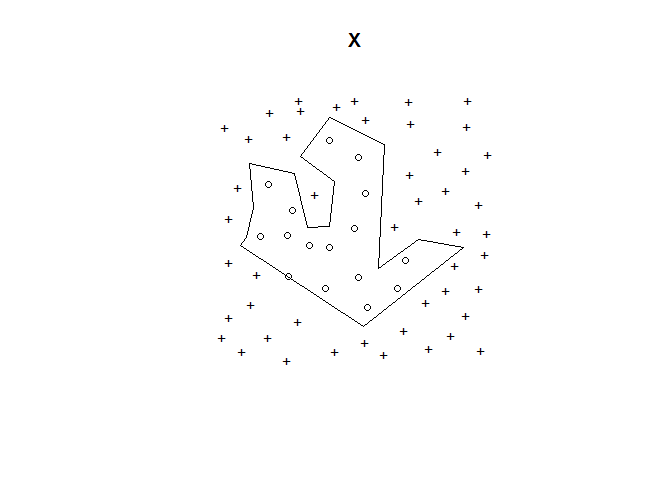## Video: Create a spatstat point pattern with a polygonal window (study region)

### Marked point patterns

Points in a spatial point pattern may carry additional information called a ‘mark’. A mark can represent additional information like height, diameter, species, etc. It is important to know that marks are not covariates (the points are not a result of the mark values!).

``````#from the spatstat help:
# marks
m <- sample(1:2, 20, replace=TRUE)
m <- factor(m, levels=1:2)
X <- ppp(x, y, c(0,1), c(0,1), marks=m)
``````

``````plot(X)
``````With Bio7 and ImageJ marked point patterns can be created with the ‘Selection’ action which can transfer a SpatialPointDataFrame which again can be converted to a marked point pattern.

1. Select points and transfer the selections to the ROI Manager
2. Measure the selections and transfer the ImageJ Results Table to R with the Image-Methods view ‘IJ RT’ action
3. Transfer the points of the ROI Manager as a SpatialPointsDataFrame (Enable the ‘Add selected data frame’ option and select the dataframe in the combobox)
4. Convert the SpatialPointsDataFrame to a spatstat point pattern object with the results table as marks

``````library(maptools)
library(spatstat)
spatialPointsDF<-as(spatialPointsDataFrame, "ppp")
``````

``````plot(spatialPointsDF)
````````````#print a summary!
summary(spatialPointsDF)

## Marked planar point pattern:  8 points
## Average intensity 0.0002494232 points per square unit
##
## Coordinates are integers
## i.e. rounded to the nearest unit
##
## Mark variables: Area, Mean, Min, Max, X, Y
## Summary:
##       Area        Mean          Min           Max            X
##  Min.   :0   Min.   :160   Min.   :160   Min.   :160   Min.   : 75.0
##  1st Qu.:0   1st Qu.:190   1st Qu.:190   1st Qu.:190   1st Qu.:124.9
##  Median :0   Median :220   Median :220   Median :220   Median :198.5
##  Mean   :0   Mean   :209   Mean   :209   Mean   :209   Mean   :173.1
##  3rd Qu.:0   3rd Qu.:232   3rd Qu.:232   3rd Qu.:232   3rd Qu.:221.0
##  Max.   :0   Max.   :248   Max.   :248   Max.   :248   Max.   :233.5
##        Y
##  Min.   : 27.50
##  1st Qu.: 63.25
##  Median :119.25
##  Mean   :129.06
##  3rd Qu.:204.38
##  Max.   :230.00
##
## Window: rectangle = [75, 233] x [27, 230] units
## Window area = 32074 square units
``````

You can also transfer a dataframe from the Table view of Bio7 (rows must be equal to the number of points!) and assign it to the point pattern.

## Video: Create a spatstat marked point pattern:

### Covariates

Tropical rainforest point pattern dataset bei. We want to find out if trees prefer a steep or flat terrain. The data consists of extra covariate data in ‘bei.extra’, which contains a pixel image of terrain elevation and a pixel image of terrain slope.

``````data(bei)
par(mfrow = c(1, 2))
``````

``````plot(bei)
````````````plot(slope)
``````### Exploratory data analysis

``````data(bei)
b <- quantile(Z, probs = (0:4)/4)
Zcut <- cut(Z, breaks = b, labels = 1:4)
V <- tess(image = Zcut)
plot(V)
plot(bei, add = TRUE, pch = "+")
``````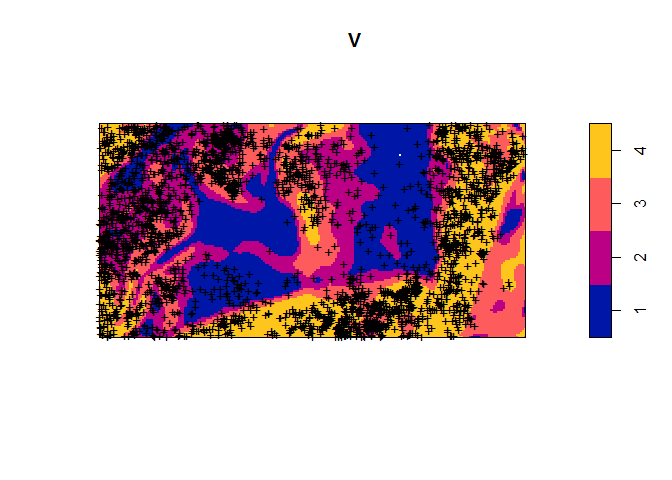Tesselated:

``````qb <- quadratcount(bei, tess = V)
plot(qb)
``````The plot below is an estimate of the intensity p(z) as a function of terrain slope z. It indicates that the Beilschmiedia trees are relatively unlikely to be found on flat terrain (where the slope is less than 0.05) compared to steeper slopes.

``````plot(rhohat(bei, slope))
``````The study region is divided into rectangles (quadrats) of equal size, and the number of points in each rectangle is counted.

``````Q <- quadratcount(X, nx = 4, ny = 3)
Q

##                x
## y               [0,0.25] (0.25,0.5] (0.5,0.75] (0.75,1]
##   (0.667,1]            0          2          1        3
##   (0.333,0.667]        2          2          2        1
##   [0,0.333]            0          1          2        4
``````

``````plot(X)
plot(Q, add = TRUE, cex = 2)
``````### Ripley’s K function

``````K <- Kest(X)
plot(K)
``````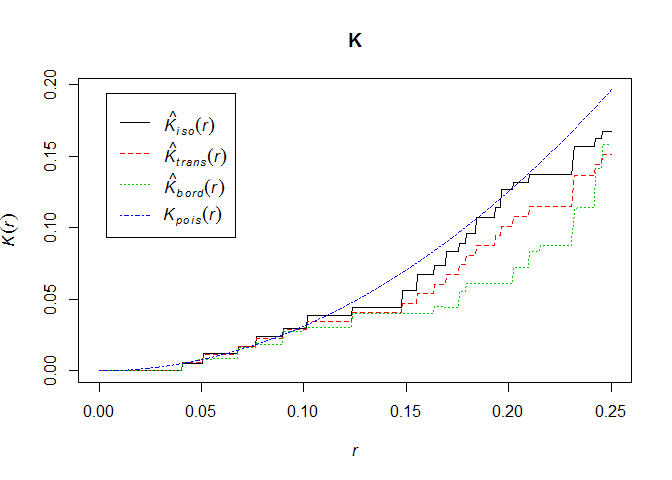### L function (common linear transformation of K)

• lines below theoretical line -> over-dispersion
• lines above theoretical line -> aggregation

``````L <- Lest(X)
plot(L, main = "L function")
``````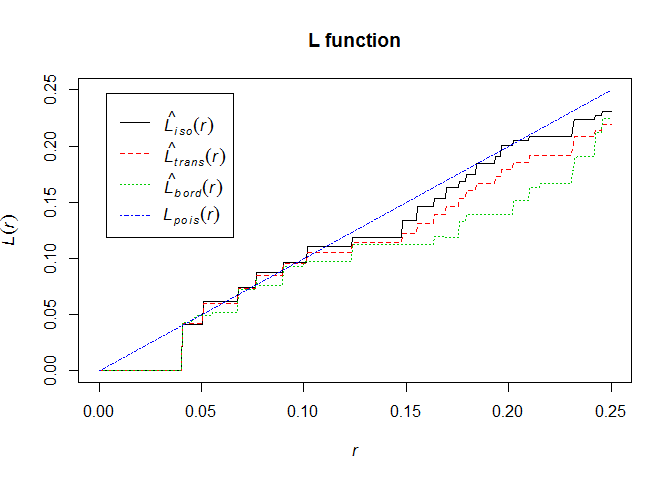### Generate an envelope for the K and L function

``````ENV <- envelope(Y = swedishpines, fun = Kest, nsim = 40)

## Generating 40 simulations of CSR  ...
## 1, 2, 3, 4, 5, 6, 7, 8, 9, 10, 11, 12, 13, 14, 15,
## 16, 17, 18, 19, 20, 21, 22, 23, 24, 25, 26, 27, 28, 29, 30,
## 31, 32, 33, 34, 35, 36, 37, 38, 39,  40.
##
## Done.
``````

``````plot(ENV)
````````````ENVL <- envelope(Y = swedishpines, fun = Lest, nsim = 40)

## Generating 40 simulations of CSR  ...
## 1, 2, 3, 4, 5, 6, 7, 8, 9, 10, 11, 12, 13, 14, 15,
## 16, 17, 18, 19, 20, 21, 22, 23, 24, 25, 26, 27, 28, 29, 30,
## 31, 32, 33, 34, 35, 36, 37, 38, 39,  40.
##
## Done.
``````

``````plot(ENVL)
``````### Creation of line patterns

``````linePattern <- psp(runif(10), runif(10), runif(10), runif(10), window=owin())
plot(linePattern)
``````## Video: Create a spatstat line pattern

### Dirichlet Tessellation of point pattern

``````X <- runifpoint(42)
plot(dirichlet(X))
``````### Point patterns in 3D

``````threeDPpp <- pp3(runif(10), runif(10), runif(10), box3(c(0,1)))
plot(threeDPpp)
``````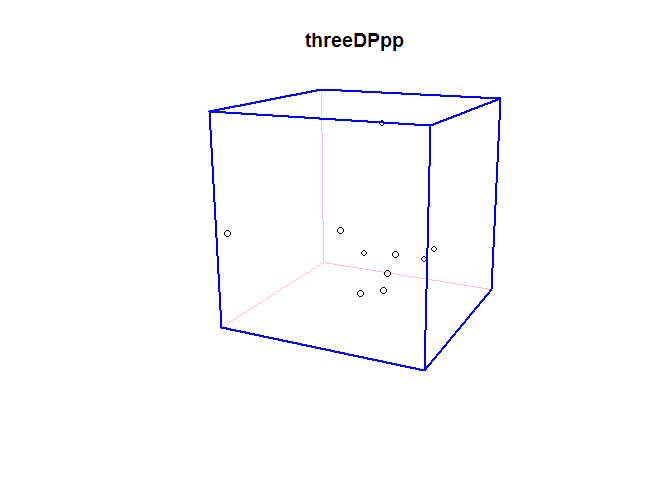## Video: Create a 3D point pattern from SpatialPoints measurements

### Nearest neighbour measurements in 3D

``````nndist(threeDPpp)

##   0.3721581 0.1554657 0.2885439 0.1554657 0.5179962 0.6377469 0.1899220
##   0.2733618 0.1785442 0.1785442
``````

### Ripley’s K in 3D

``````threeDPppKest <- K3est(threeDPpp)
plot(threeDPppKest)
``````### Point pattern example on a Linear Network (e.g. car accidents on a road)

``````data(simplenet)
Xlin <- rpoislpp(5, simplenet)
plot(Xlin)
``````### Fit a point process model to an observed point pattern.

``````# fit the stationary Poisson process
# to point pattern 'nztrees'
data(nztrees)
fitted<-ppm(nztrees)
modelFitted<-rmh(fitted)

## Extracting model information...Evaluating trend...done.
## Checking arguments..determining simulation windows...
``````

``````plot(modelFitted, main="Fitted Model")
``````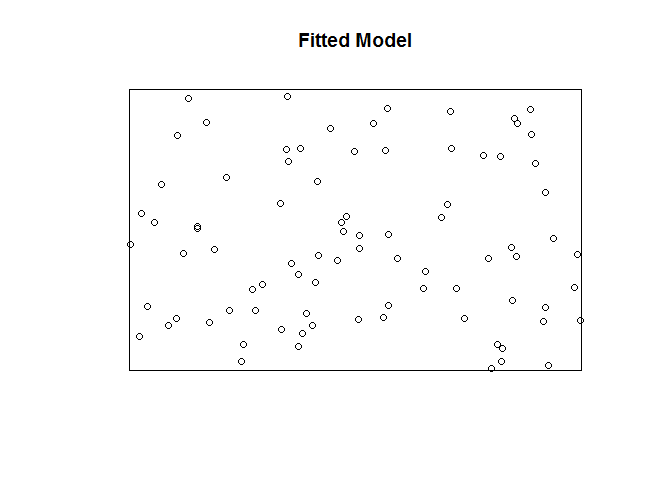### References

Used for this script:

(1) A. Baddeley and R. Turner. Spatstat: an R package for analyzing spatial point patterns Journal of Statistical Software 12: 6 (2005) 1-42. www.jstatsoft.org ISSN: 1548-7660

(2) A. Baddeley and R. Turner. Modelling spatial point patterns in R. Chapter 2, pages 23-74 in In Case Studies in Spatial Point Pattern Modelling (eds. A. Baddeley, P. Gregori, J. Mateu, R. Stoica and D. Stoyan) Lecture Notes in Statistics 185. New York: Springer-Verlag 2006. ISBN: 0-387-28311-0

(3) A. Baddeley. Analysing Spatial Point Patterns in R. Workshop Notes, December 2010. Published online by CSIRO, Australia. Download here (232 pages, pdf, 12.2Mb)